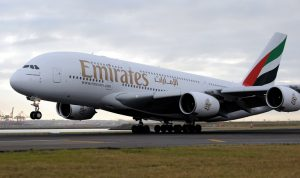# Number quizThis number quiz gives students a chance to use numbers other than their age and the date which is all that is usually required of them. The answers to these questions range from very small to very large numbers.

Divide the class into teams and ask the question, the team with the closest answer gets the point. Make sure you start with a different team each round because the first team to answer has a disadvantage.

If there are only two teams then you can play over and under. The first team gives an answer and the second team must simply decide whether the answer is over or under the other team’s answer.

Q How far away is the sun?
149.6 million km

What is the population of Canada?
35.15 million (2013)

How many kilometres in a mile?
1.6

How many holes in a standard round of golf?
18

How high is the Eiffel Tower?
300 meters

What is the square root of 144
12

What is nine squared?
81

How many astronauts have walked on the moon?
12

How long is the great wall of China?
21,196 km

What is the maximum number of passengers an Airbus A380 can carry?
853

What is the speed of sound?
340.39 meters a second, 1,236 km an hour

What is the value of Pi? (whoever gets the most digits right wins)
3.1415926535897932384626433832795

How many states in the USA?
50